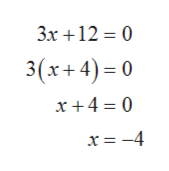11:52mathxl.com2.6.65Find the horizontal and vertical asymptotes then graph the rational functiony3x 12If there is a horizontal asymptote, what is it? Select the correct choice below and fill in any answer boxes within your choice.O A. The horizontal asymptote is(Type an equation.)O B. There is no horizontal asymptote.

Question

Can you help me step by step with this problem?help_outlineImage Transcriptionclose11:52 mathxl.com 2.6.65 Find the horizontal and vertical asymptotes then graph the rational function y 3x 12 If there is a horizontal asymptote, what is it? Select the correct choice below and fill in any answer boxes within your choice. O A. The horizontal asymptote is (Type an equation.) O B. There is no horizontal asymptote. fullscreen
Step 1

Let the given function is y=4/(3x+12).

The graph of y has vertical asymptotes at those values of x at which the denominator is equal to zero.

Hence the given function has vertical asymptotes at:help_outlineImage Transcriptionclose3x 12 0 3(x+4)0 x +4 0 x= -4 fullscreen
Step 2

Thus, the graph will have vertical asymptotes at x = - 4 line.

Step 3

To find the horizontal asymptote, if the degree of denominator is greater than the degree of numerator then the graph of the function will have a horizontal asym...

Want to see the full answer?

See Solution

Want to see this answer and more?

Our solutions are written by experts, many with advanced degrees, and available 24/7

See Solution
Tagged in

Other